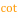# Cotangent

 Block Group: Trigonometric Functions Icon:The Cotangent block returns the cotangent of an angle, in degrees.

For information on using dataflow blocks, see Dataflow.

## Input/Output Property

The following property of the Cotangent block can take input and give output.

• input (number)

input specifies the angle to get the cotangent for, in degrees.

## Output Property

The following property of the Cotangent block can give output but cannot take input.

• output (number)

output returns the cotangent of the input.

## Example

The following image shows an example of the Cotangent block. In this example, the angle is 30 degrees, and the cotangent is 1.732.